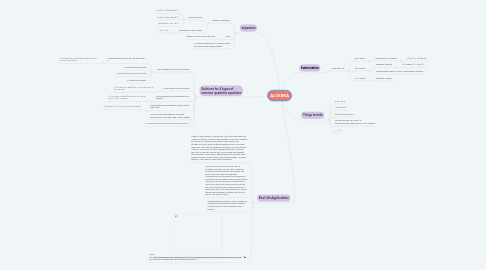# ALGEBRANATANIA LEOW EN-QI 1G
Get Started. It's FreeALGEBRA## 1. expansion

### 1.1. algebraic identities

1.1.1. perfect square

1.1.1.1. (a+b)^2 =a^2+2ab+b^2

1.1.1.2. (a-b)^2 =a^2-2ab+b^2

1.1.1.3. (a-b)(a+b) = a^2 - b^2

1.1.2. difference of two squares

1.1.2.1. a^2 - b^2

### 1.2. FOIL

1.2.1. stands for First Outer Inner Last

## 2. factorisation

### 2.1. 1. Take out HCF

2.1.1. 2a) 2 terms

2.1.1.1. Difference of 2 squares

2.1.1.1.1. a^2-b^2 = (a-b)(a+b)

2.1.2. 2b) 3 terms

2.1.2.1. Algebraic Identity

2.1.2.1.1. a^2+2ab+b^2 = (a+b)^2

2.1.2.2. Multiplication frame or Cross-Multiplication method

2.1.3. 2c) 4 terms

2.1.3.1. Grouping Method

## 4. Solutions for 4 types of common quadratic equations

### 4.1. 3 terms without any common factor

4.1.1. 1. ensure expression on the left hand side = 0

4.1.1.1. 1. x^2-4x-5=0 2. (x-5)(x+1)=0 3. x-5=0 or x+1=0 4. x=5 or x=-1

4.1.2. 2. factorise left hand side

4.1.3. 3. Apply "Zero Product Principle"

4.1.4. 4. Solve the equation

### 4.2. terms with a common factor

4.2.1. 1. x^2-5x=0 2. x(x-5)=0 3. x=0 or x-5=0 4. x=0 or x=5

### 4.3. 2 terms which involve difference of 2 squares

4.3.1. 1. x^2-49=0 2. (x+7)(x-7)=0 3. x+7=0 or x-7+0 4. x=-7 or x=7

### 4.4. 2 terms which involve taking square root on both sides

4.4.1. 1. x^2-25=0 2. x^2=25 3. x=±√25 x=±5